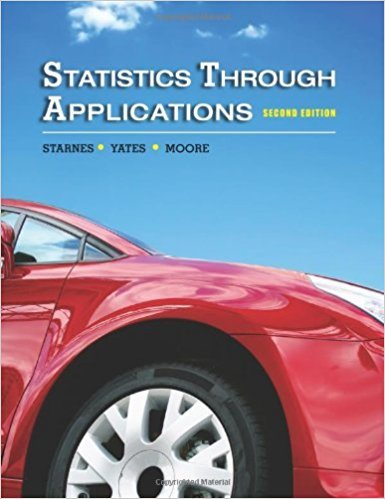×
Get Full Access to Statistics Through Applications - 2 Edition - Chapter 2 - Problem 2.24
Get Full Access to Statistics Through Applications - 2 Edition - Chapter 2 - Problem 2.24

×

# Travel time Th e dotplot on the facing page displays dataISBN: 9781429219747 133

## Solution for problem 2.24 Chapter 2

Statistics Through Applications | 2nd Edition

• Textbook Solutions
• 2901 Step-by-step solutions solved by professors and subject experts
• Get 24/7 help from StudySoup virtual teaching assistantsStatistics Through Applications | 2nd Edition

4 5 1 347 Reviews
19
5
Problem 2.24

Travel time Th e dotplot on the facing page displays data on students responses to the question How long does it usually take you to travel to school? (a) Make a well-labeled histogram of the data. (b) Describe the shape, center, and spread of the distribution. Are there any outliers?

Step-by-Step Solution:
Step 1 of 3

Lab #1 The Penny Problem: Review The Scientific Method Scientists use the scientific method to answer questions and provide explanations about natural phenomena. It is a logical process based on careful observation and experimentation. 1. Observation: leads to a question and discussion about the observed phenomena. 2. Hypothesis: based on the observation scientists generate a hypothesis or tentative explanation for the observed phenomena. Hypothesis are written as two statements; one called the null hypothesis, and the other called alternate hypotheses. - The Null Hypothesis – is written in a way that shows no difference between the groups under study. Ex: There is statistically significant relationship between

Step 2 of 3

Step 3 of 3

##### ISBN: 9781429219747

The answer to “Travel time Th e dotplot on the facing page displays data on students responses to the question How long does it usually take you to travel to school? (a) Make a well-labeled histogram of the data. (b) Describe the shape, center, and spread of the distribution. Are there any outliers?” is broken down into a number of easy to follow steps, and 50 words. This textbook survival guide was created for the textbook: Statistics Through Applications, edition: 2. Since the solution to 2.24 from 2 chapter was answered, more than 369 students have viewed the full step-by-step answer. This full solution covers the following key subjects: data, Travel, make, describe, displays. This expansive textbook survival guide covers 10 chapters, and 710 solutions. Statistics Through Applications was written by and is associated to the ISBN: 9781429219747. The full step-by-step solution to problem: 2.24 from chapter: 2 was answered by , our top Statistics solution expert on 11/10/17, 06:04PM.

Unlock Textbook Solution